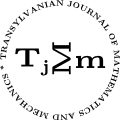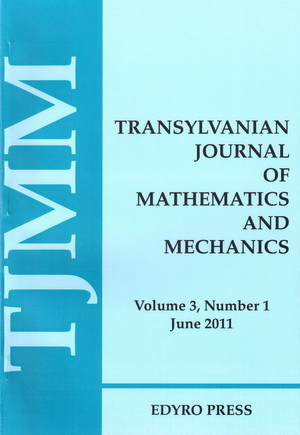ISSN: 2067-239X
ISSN(on-line): 2067-239X

Indexed in:
Mathematical Reviews
Zentralblatt MATH
EBSCO

Front cover# Volume 5 (2013), Number 1

## Stability in Nonlinear Neutral Difference Equations with Variable Delays

### Author(s): ABDELOUAHEB ARDJOUNI and AHCENE DJOUDI

Abstract: In this paper we use fixed point method to prove asymptotic stability results of the zero solution of a nonlinear neutral difference equation with variable delays. An asymptotic stability theorem with a sufficient condition is proved. The obtained results improve and generalize those due to Raffoul (2006), Yankson (2009) and Islam and Yankson (2005).

## Subclasses of p-valent Functions Defined by Convolution Involving the q-hypergeometric Function

### Author(s): HUDA ALDWEBY and MASLINA DARUS

Abstract: Motivated by the familiar $q$-hypergeometric functions, we introduce here two general subclasses ${S}_{\lambda ,\mu ,\eta }^{p,r,s}\left({a}_{i},{b}_{j},q,\alpha ,\beta \right)$ and ${C}_{\lambda ,\mu ,\eta }^{p,r,s}\left({a}_{i},{b}_{j},q,\alpha ,\beta \right)$ of analytic $p$-valent functions with negative coefficients in the unit disk. These classes generalize well known classes of starlike and convex functions. For these classes, some results which include the coefficient estimates, the integral means inequalities and $\nu$-$\rho$-neighborhood are obtained.

## Some Applications of Fejér’s Inequality for Convex Functions (II)

### Author(s): S.S. DRAGOMIR and I. GOMM

Abstract: Some applications of Fejér’s inequality for convex functions are explored. Upper and lower bounds for the weighted integral ${\int }_{a}^{b}{\left(x-\frac{a+b}{2}\right)}^{2}f\left(x\right)dx$ under various assumptions for $f$ with applications to the trapezoidal quadrature rule are given. Some inequalities for special means are derived.

## Extension of the Bertrand Theorem to the Relativistic Classical Mechanics

### Author(s): LAUREAN HOMORODEAN

Abstract: A new approach of the relativistic motion of a particle in a central field is presented. It is based on a modified form of the Hamilton function of the particle. This form allows us to extend the Bertrand theorem from the non-relativistic classical mechanics to the relativistic classical mechanics.

## Generalized Growth of Harmonic Functions in Hyper Spheres

### Author(s): DEVENDRA KUMAR and RAJBIR SINGH

Abstract: The paper deals with growth of functions which are harmonic in seve ral variables. The type and lower type with respect to proximate order have been characterized in terms of derivatives of functions harmonic in the neighborhood of origin in ${ℝ}^{n}$. Our results generalize some results of Fryant and Shankar.

## Laplace Transform Technique for Pseudoparabolic Equation with Nonlocal Conditions

### Author(s): AHCENE MERAD and ABDELFATAH BOUZIANI

Abstract: In this paper we present a Laplace transform technique for solving the pseudoparabolic equation with nonlocal (integral) conditions. A Laplace transform technique is described for the solution of the considered equation. Finally, we obtain the solution using a numerical technique by inverting the Laplace transform.

## Composition Operators on Cesaro-Orlicz Sequence Spaces

### Author(s): KULDIP RAJ, SEEMA JAMWAL and SUNIL K. SHARMA

Abstract: In this paper we characterize the boundedness, invertibility and Fredholmness of composition operators on the Cesaro-Orlicz sequence spaces Ces${}_{\phi }$.

## Some Integral Inequalities of Hermite-Hadamard Type for Extended $\left(s,m\right)$-convex Functions

### Author(s): BO-YAN XI, YAN WANG and FENG QI

Abstract: In the paper, the authors establish some new integral inequalities of Hermite-Hadamard type for extended $\left(s,m\right)$-convex functions.